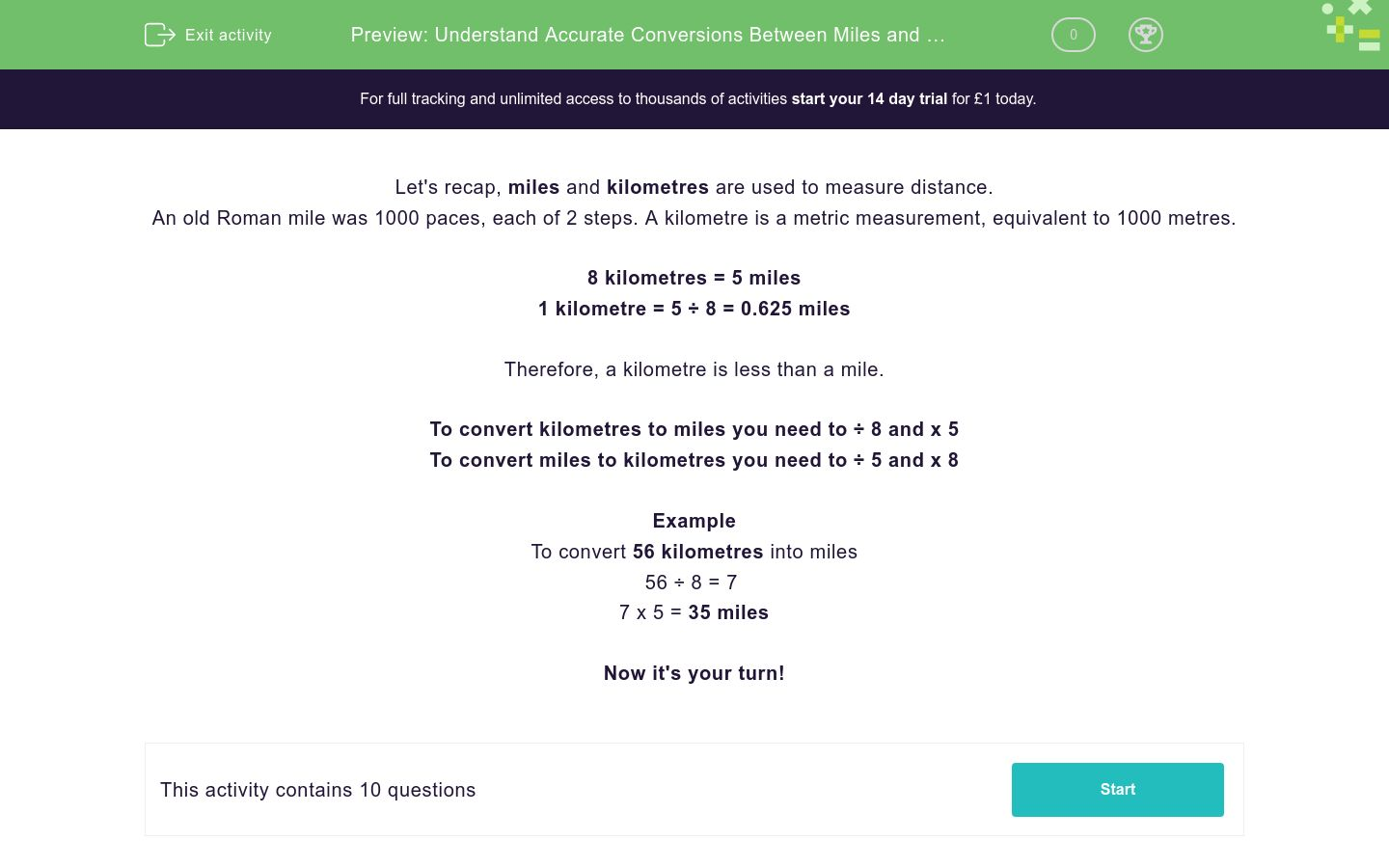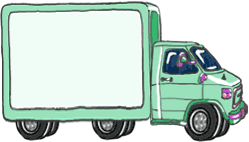# Understand Accurate Conversions Between Miles and Kilometres

In this worksheet, students will use the units of miles and kilometres to complete a variety of conversions.Key stage:  KS 2

Curriculum topic:   Measurement

Curriculum subtopic:   Convert Miles/Kilometres

Difficulty level:### QUESTION 1 of 10

Let's recap, miles and kilometres are used to measure distance.

An old Roman mile was 1000 paces, each of 2 steps. A kilometre is a metric measurement, equivalent to 1000 metres.

8 kilometres = 5 miles

1 kilometre = 5 ÷ 8 = 0.625 miles

Therefore, a kilometre is less than a mile.

To convert kilometres to miles you need to ÷ 8 and x 5

To convert miles to kilometres you need to ÷ 5 and x 8

Example

To convert 56 kilometres into miles

56 ÷ 8 = 7

7 x 5 = 35 miles

The distance from London to Glasgow is 415 miles.

Convert this into kilometres.

A lorry driver has undertaken a lot of journeys in 7 days.

He has covered a distance of 624 km.

Can you convert this into miles?A racetrack is 4.5 miles long.

What would the length be in km?7.0 km

72 km

0.72 km

7.2 km

A racetrack is 3.2 km long.

What would the length be in km?

2.0 miles

2.2 miles

1.8 miles

2.1 miles

A racetrack is 7.5 km long.

What would the length be in miles?

5.4 miles

4.5 miles

4.2 miles

4.6 miles

A racetrack is 7.9 miles long.

What would the length be in kilometres?

12.5 km

12.64 km

12.45 km

12.04 km

Can you match the distance in miles with the equivalent distance in kilometres?

## Column B

5 miles
3.2 km
4 miles
8 km
2 miles
6.4 km
6 miles
9.6 km

How many miles are there in 2 kilometres?

How many kilometres are there in 10 miles?

A can of cola is 10 cm high.

If the cans were laid end to end, how many cans would be needed to cover a distance of 1 mile?• Question 1

The distance from London to Glasgow is 415 miles.

Convert this into kilometres.

EDDIE SAYS
Remember, the rule to convert miles to kilometres is ÷ 5 and x 8 415 ÷ 5 = 83 83 x 8 = 664 km
• Question 2

A lorry driver has undertaken a lot of journeys in 7 days.

He has covered a distance of 624 km.

Can you convert this into miles?EDDIE SAYS
Remember in order to convert km to miles, we ÷ 8 and then x 5. 624 ÷ 8 = 78 78 x 5 = 390 miles
• Question 3

A racetrack is 4.5 miles long.

What would the length be in km?7.2 km
EDDIE SAYS
This was a tricky one as we had to convert a decimal number. 4.5 miles ÷ 5 = 0.9 0.9 x 8 = 7.2 km
• Question 4

A racetrack is 3.2 km long.

What would the length be in km?

2.0 miles
EDDIE SAYS
Again, this question requires that we calculate carefully with decimal numbers. 3.2 km ÷ 8 = 0.4 0.4 x 5 = 2.0 miles
• Question 5

A racetrack is 7.5 km long.

What would the length be in miles?

4.5 miles
EDDIE SAYS
7.2 km ÷ 8 = 0.9 0.9 x 5 = 4.5 miles
• Question 6

A racetrack is 7.9 miles long.

What would the length be in kilometres?

12.64 km
EDDIE SAYS
7.9 miles ÷ 5 = 1.58 1.58 x 8 = 12.64 km Great focus, keep it up! You're past halfway in the activity.
• Question 7

Can you match the distance in miles with the equivalent distance in kilometres?

## Column B

5 miles
8 km
4 miles
6.4 km
2 miles
3.2 km
6 miles
9.6 km
EDDIE SAYS
To correctly match up the number of miles to the corresponding kilometres, we need to ÷ 5 then x 8. 5 miles = 8 km 4 miles = 6.4 km 2 miles = 3.2 km 6 miles = 9.6 km
• Question 8

How many miles are there in 2 kilometres?

1.25 miles
1.25
EDDIE SAYS
Remember to convert kilometres to miles, we have to ÷ 8 and then x 5. 2 ÷ 8 = 0.25 0.25 x 5 = 1.25 miles This should be like second nature to you now!
• Question 9

How many kilometres are there in 10 miles?

16 km
16
EDDIE SAYS
The rule to convert miles to kilometres is ÷ 5 and then x 8. So, 10 ÷ 5 = 2 2 x 8 = 16 km
• Question 10

A can of cola is 10 cm high.

If the cans were laid end to end, how many cans would be needed to cover a distance of 1 mile?EDDIE SAYS
To work through this problem, we have to work out the distance different amounts of cans could cover. 1 can = 10 cm Remember there are 100 cm in 1 metre therefore 10 cans = 1 metre Remember there are 1000 metres in 1 km therefore 10,000 cans = 1 km So to work out the number of cans in 1 mile, we need to multiply 10,000 by 1.6 as 1.6 km = 1 mile 10,000 x 1.6 = 16,000 16,000 cans covers 1 mile Great focus, that’s another activity completed!
---- OR ----

Sign up for a £1 trial so you can track and measure your child's progress on this activity.

### What is EdPlace?

We're your National Curriculum aligned online education content provider helping each child succeed in English, maths and science from year 1 to GCSE. With an EdPlace account you’ll be able to track and measure progress, helping each child achieve their best. We build confidence and attainment by personalising each child’s learning at a level that suits them.

Get started